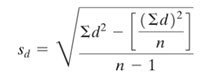# What are d-bar and s sub d?This question comes up frequently in my intro stats class. We are covering paired (dependent) samples hypothesis tests and the explanation in the textbook gives the students a fuzzy discussion and includes a complex formula for finding the latter.

“d-bar” (my editor is not letting me use the correct symbol, but this is the letter “d” with a bar over it) is just the average of the differences (d) in the two samples.

“s sub d” (editor again) is just the plain old standard deviation of the differences, despite this rather complex formula:Here is an example of how to find them using basic Excel functions:

Here is the Excel worksheet with formulas:Unfortunately, StatCrunch does not give you “s sub d” directly in any of the built-in analyses and but you can find them both by the following method:

### 1 thought on “What are d-bar and s sub d?”

1.that was very help. thank you !!!
\

This site uses Akismet to reduce spam. Learn how your comment data is processed.